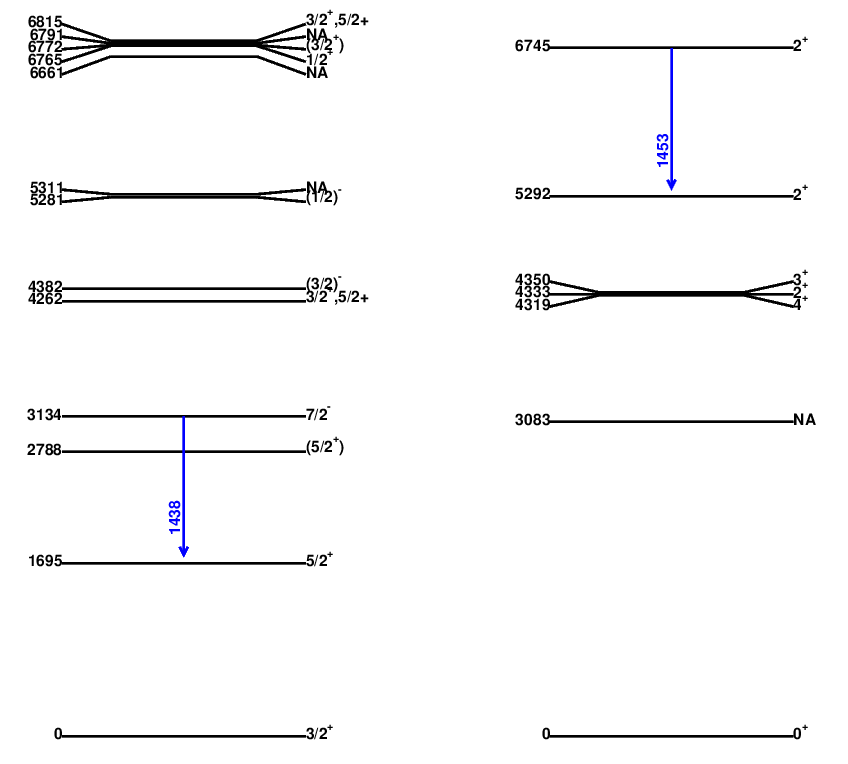# 'highlighter' Program Introduction

highlighter is an application based on lvl_builder program. Sometimes, we just want to plot a subset of the levels and gammas or to examine the coincidence relation, then it is good to use highlighter program.

# How to run

highlighter is a python script. Just type in `python ./highlighter.py [level_gam_data ] `,
where the level_gam_data file follows the format for lvl_builder program (i.e. having @lvlE 0 @bandN 1 etc...) You can use your own level_gam_data file, or the nndc_result.txt file from parseNNDC2 program.

Please see my Github (link) for the files.Since highlighter will use lvl_builder program, and so I put it in the same folder as lvl_builder. You will need to download the entire folder.

highlighter is in a menu-driven fashion. The following is the main menu when you enter the program.
I use nndc_result.txt as my data file ( ./highlighter.py nndc_result.txt)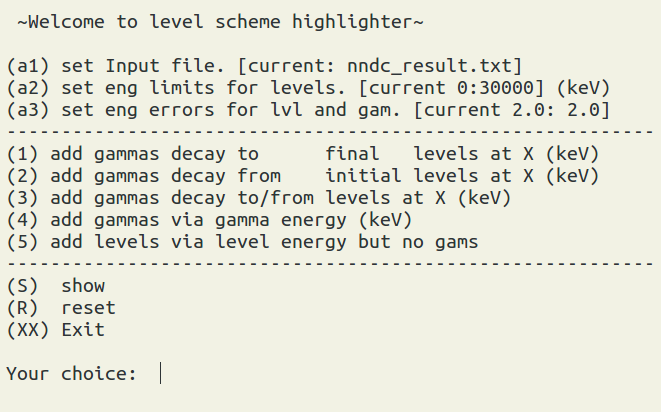# Setting

The setting options are a1, a2, and a3:
"a1" option allows you to change the input source file.

"a2" option allows you to set the lower and upper level energy limits. by default, it is 0 to 30 MeV.

"a3" options allows you to set the errors ( selection range). For example, when you add a gamma at 500 keV, actually, the program will search the gams whose energies are between 500 - w_g to 500+ w_g, where w_g is the gamma error, which is 2 keV by default. Moreover, if we want to select states at 1 +- 0.5 MeV and 3 +- 0.5 MeV. And then we just need to set the level error to be 500.

# Selections

There are 5 section options, and they are all independent.

Example1: add gammas decay to final levels at X (keV)
Suppose in your data, there are gammas from 31Si and 26Mg., let's check what are the gammas that decay directly to the ground states of 31Si and 26Mg. To do this, we use option 1, and type in 0 for ground state. And then type "x" to get back to the main menu, and type "s" to show.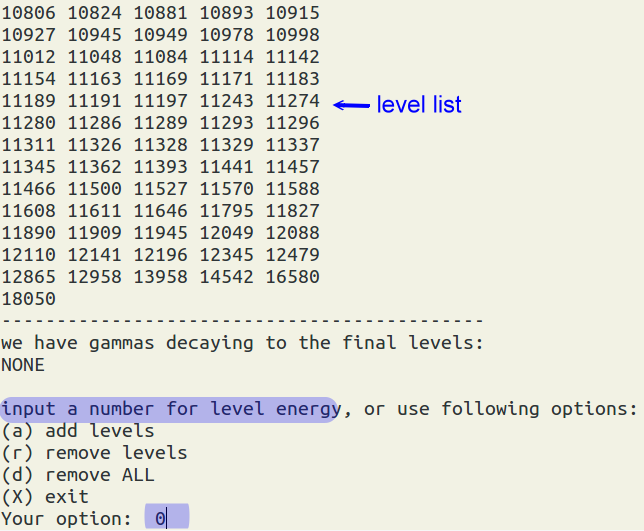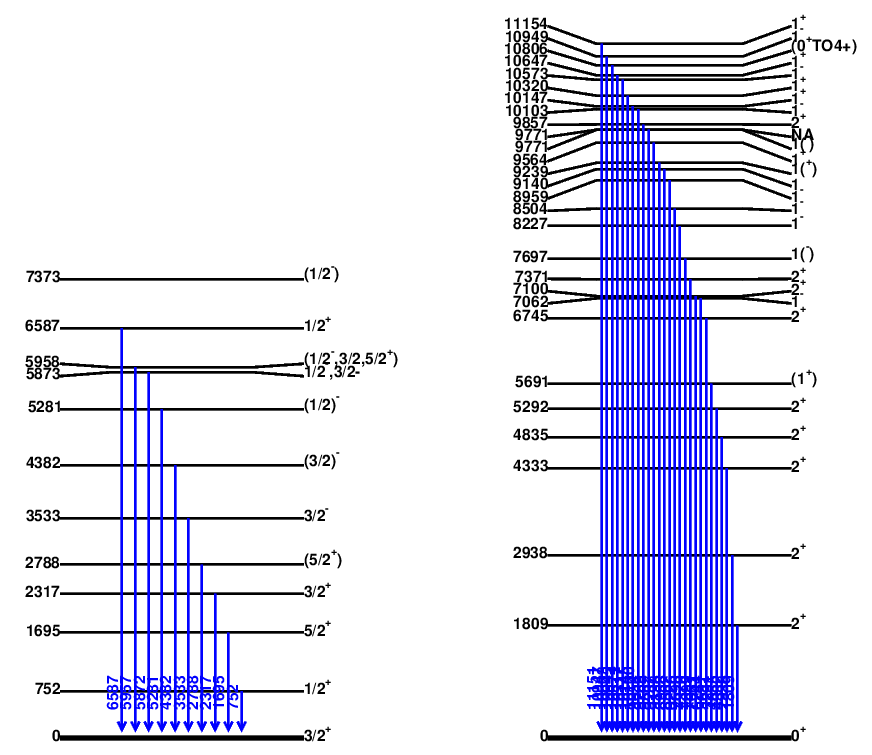Example2: add gammas decay from initial levels at X (keV)
For the next test, we want to see what are the gammas decaying from 4300 +- 100 keV states (just for fun). First type "R" in the main menu to reset our previous selections. and type in "a3", and input "100,2" for the erros for levels and gammas. Use (2) to add the gammas decay from initial levels at X (keV). Input 4300. use (x) to go back the main menu, and type "s" to show.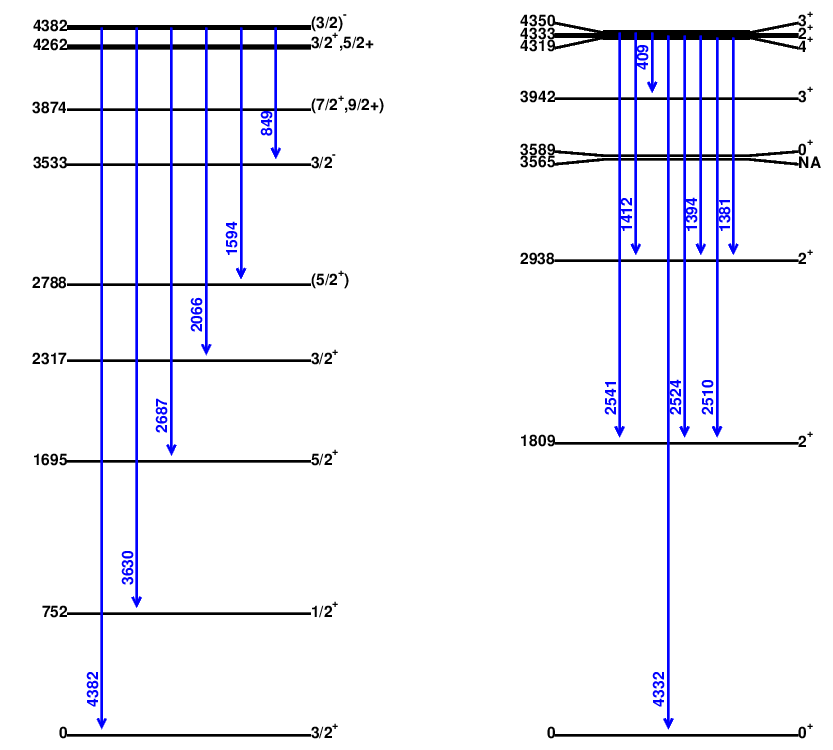Example3: add gammas decay to/from levels at X (keV)
option "3" is the combination of "1" and "2" options. Let's reset everything, and suppose we want to see what the gammas connect to 1695+-2 keV and 2788+-2 keV states. we use option (3), then (a), then type in "1695 2788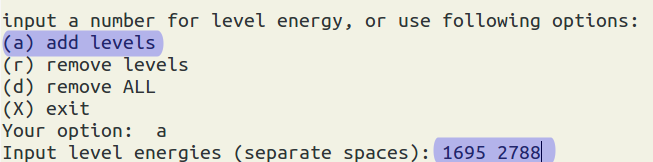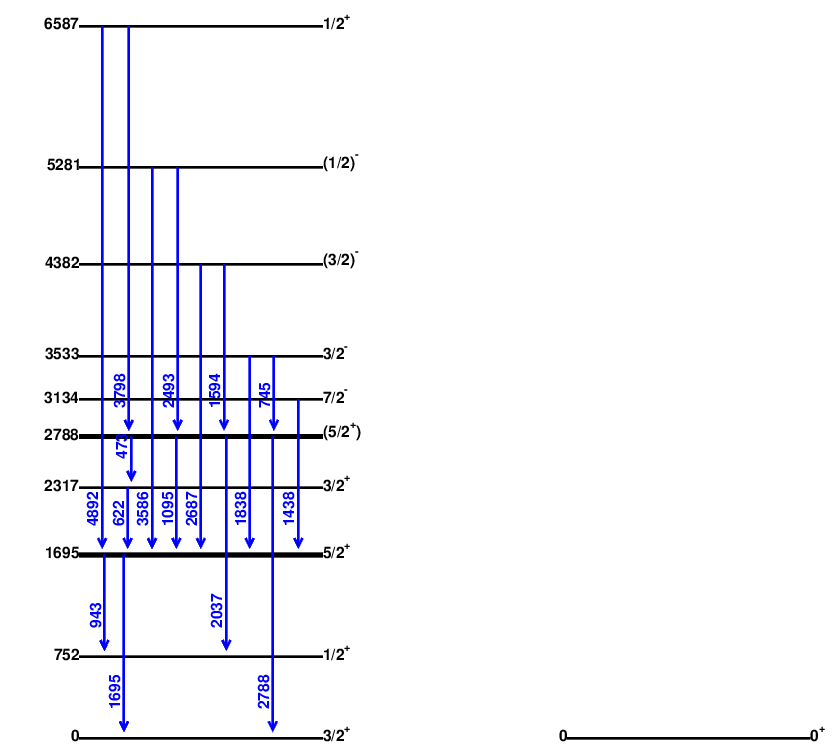Example4: add gammas via gamma energy (keV)
Suppose we want to see whether we have gammas near the energy 1439 +- 20 keV .
First let's reset everything., we use (a3) to adjust our errors (gam energy selection range ) to 20 keV. Then use (4), and type in 1439, and then use (x) to go back, and then (s) to show.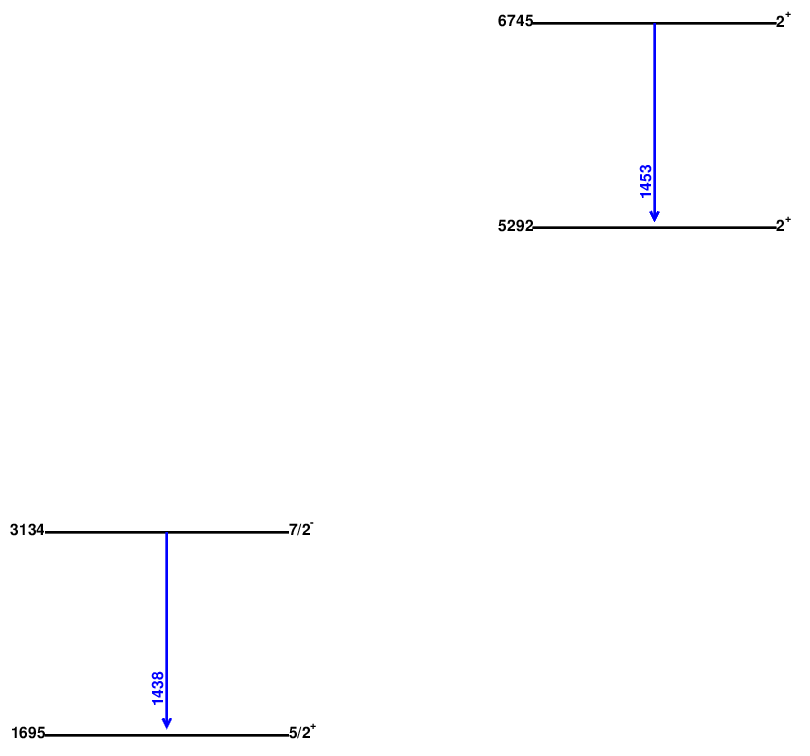Example5: add levels via level energy but no gams
Based on example 4, suppose we want to add levels at 0, 2788+-100, 4300+-100 but no gammas . It is the right time to use option 5. we use (a3) to adjust our errors to "100,20". Then use (5)-->(a), and type in "0 2788 4300, and then use (x) to go back, and then (s) to show.